# High School Math : Setting up Expressions

## Example Questions

### Example Question #1 : Setting Up Expressions

In April, the price of a t-shirt is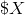.   In May, the store increases the price by 50%, so that the new price is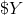. Then in June, the store decreases the price by 50%, so that the t-shirt price is now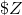.  What is the ratio of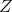to?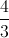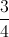Explanation:

If the original price of the T-shirt is, increasing the price by 50% means that the new price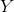is 150% of, or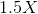.

If the price is then decreased by 50%, the new priceis 50% ofor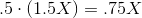The ratio oftois then: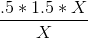The's in the numerator and denominator cancel, leaving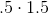, or.